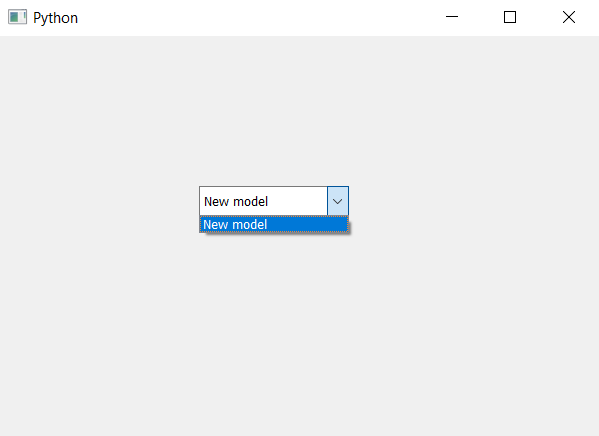Related Articles

# PyQt5 – How to add model to ComboBox

• Last Updated : 22 Apr, 2020

In this article we will see how we can set model to the combo box. Model is used to set the items of the combo box, in order to set the model we will use `setModel` method.

Note : If we add model after the insertion of items it will clear all the elements so model should be added before any insertion of elements

Syntax : combo_box.setModel(model)

Argument : It takes QStandardItemModel object as argument

Return : None

Below is the implementation –

 `# importing libraries``from` `PyQt5.QtWidgets ``import` `*` `from` `PyQt5 ``import` `QtCore, QtGui``from` `PyQt5.QtGui ``import` `*` `from` `PyQt5.QtCore ``import` `*` `import` `sys`` ` ` ` `class` `Window(QMainWindow):`` ` `    ``def` `__init__(``self``):``        ``super``().__init__()`` ` `        ``# setting title``        ``self``.setWindowTitle(``"Python "``)`` ` `        ``# setting geometry``        ``self``.setGeometry(``100``, ``100``, ``600``, ``400``)`` ` `        ``# calling method``        ``self``.UiComponents()`` ` `        ``# showing all the widgets``        ``self``.show()`` ` `    ``# method for widgets``    ``def` `UiComponents(``self``):`` ` `        ``# creating a combo box widget``        ``self``.combo_box ``=` `QComboBox(``self``)`` ` `        ``# setting geometry of combo box``        ``self``.combo_box.setGeometry(``200``, ``150``, ``150``, ``30``)`` ` `        ``# geek list``        ``geek_list ``=` `[``"Sayian"``, ``"Super Saiyan"``, ``"Super Sayian 2"``,``                                               ``"Super Sayian B"``]`` ` `        ``# making it editable``        ``self``.combo_box.setEditable(``True``)`` ` `        ``# adding list of items to combo box``        ``self``.combo_box.addItems(geek_list)`` ` `        ``# creating a model``        ``model ``=` `QtGui.QStandardItemModel(``0``, ``1``)`` ` `        ``# creating a item``        ``item ``=` `QtGui.QStandardItem(``"New model"``)`` ` `        ``# adding item to the model``        ``model.appendRow(item)`` ` `        ``# setting model to the combo box``        ``self``.combo_box.setModel(model)`` ` ` ` ` ` `# create pyqt5 app``App ``=` `QApplication(sys.argv)`` ` `# create the instance of our Window``window ``=` `Window()`` ` `# start the app``sys.exit(App.``exec``())`

Output :Attention geek! Strengthen your foundations with the Python Programming Foundation Course and learn the basics.

To begin with, your interview preparations Enhance your Data Structures concepts with the Python DS Course. And to begin with your Machine Learning Journey, join the Machine Learning – Basic Level Course

My Personal Notes arrow_drop_up